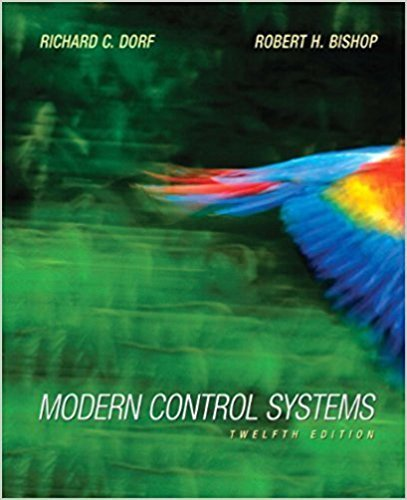×
Get Full Access to Modern Control Systems - 12 Edition - Chapter Chapter 7 - Problem E7.11
Get Full Access to Modern Control Systems - 12 Edition - Chapter Chapter 7 - Problem E7.11

×

# A robot force control system with unity feedback has a loop transfer function  Us) =ISBN: 9780136024583 453

## Solution for problem E7.11 Chapter Chapter 7

Modern Control Systems | 12th Edition

• Textbook Solutions
• 2901 Step-by-step solutions solved by professors and subject experts
• Get 24/7 help from StudySoup virtual teaching assistantsModern Control Systems | 12th Edition

4 5 1 412 Reviews
14
5
Problem E7.11

A robot force control system with unity feedback has a loop transfer function  Us) = KG(s) K(s + 2.5) (s2 + 2s + 2){s2 + 4s + 5) (a) Find the gain A' that results in dominant roots with a damping ratio of 0.707. Sketch the root locus. (b) Find the actual percent overshoot and peak time for the gain K of part (a).

Step-by-Step Solution:
Step 1 of 3

MODULE 4 ARTICLE I. INFERENCE FOR POPULATION MEANS 1) Confidence Intervals a. We can find confidence intervals of two differencesample means 2) Known Population Variances a. Let X and Y be to independent binomial random variables. b. A 100(1-α)% CI for the difference of the two meansfrom each random variable is: 3) Unknown population variance a. t-value is used 4) Large sample confidence intervals for two population proportions a. Let X and Y be to independent binomial random variables. b. Proportion p is the number of successes/n ̃ c. Let n =nx+2x d. Let p =(x+1)/ n x e. The CI is the

Step 2 of 3

Step 3 of 3

##### ISBN: 9780136024583

The full step-by-step solution to problem: E7.11 from chapter: Chapter 7 was answered by , our top Engineering and Tech solution expert on 03/16/18, 03:13PM. This full solution covers the following key subjects: . This expansive textbook survival guide covers 14 chapters, and 1211 solutions. Since the solution to E7.11 from Chapter 7 chapter was answered, more than 377 students have viewed the full step-by-step answer. Modern Control Systems was written by and is associated to the ISBN: 9780136024583. The answer to “A robot force control system with unity feedback has a loop transfer function  Us) = KG(s) K(s + 2.5) (s2 + 2s + 2){s2 + 4s + 5) (a) Find the gain A' that results in dominant roots with a damping ratio of 0.707. Sketch the root locus. (b) Find the actual percent overshoot and peak time for the gain K of part (a).” is broken down into a number of easy to follow steps, and 65 words. This textbook survival guide was created for the textbook: Modern Control Systems, edition: 12.

Unlock Textbook Solution